Question

# The three-phase wye-connected induction motor nameplate gives the following information: 400 V, 1755 rpm, 60 Hz....

The three-phase wye-connected induction motor nameplate gives the following information:
400 V, 1755 rpm, 60 Hz.
Rotor speed at no-load is 1799 rpm.
At rated voltage and frequency motor core losses are 400 W. At rated rotor speed, mechanical
losses are 400 W.
The available parameters of the motor conventional equivalent circuit (6-parameters
IEEE model) are:
R1 = 0.2 Ω, R’2 = 0.2 Ω, X1 = 0.5 Ω, X’2 = 0.2 Ω and Xφ = 20.0 Ω.
In all calculations, use the standard equivalent circuit (IEEE model 6 para-meters) of
the motor and consider that the motor operates at nominal voltage.

a) Determine the missing parameter of the motor conventional equivalent circuit (6-
parameter IEEE model). (4 points)
b) Calculate the motor no-load current. (4 points)
c) Find the no-load real power at the motor input. (2 points)
d) Determine the no-load reactive power at the motor input. (2 points)
e) Determine the current of the motor operating at rated speed. (4 points)
f) Calculate the real power at the input of the motor operating at rated speed. (2
points)
g) Determine the reactive power at the input of the motor operating at rated speed.
(2 points)
h) Find the useful mechanical power provided by the motor operating at rated
speed. (4 points)
i) Calculate the useful mechanical torque developed by the motor operating at
nominal speed. (4 points)
j) Determine the efficiency of the motor operating at rated speed. (2 points)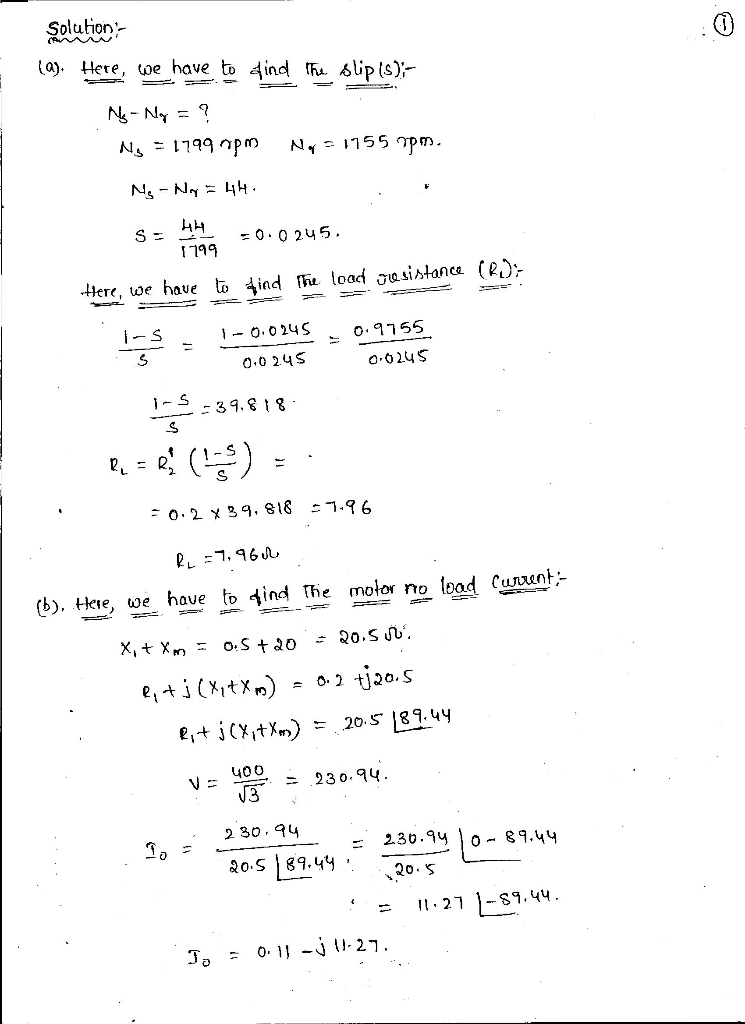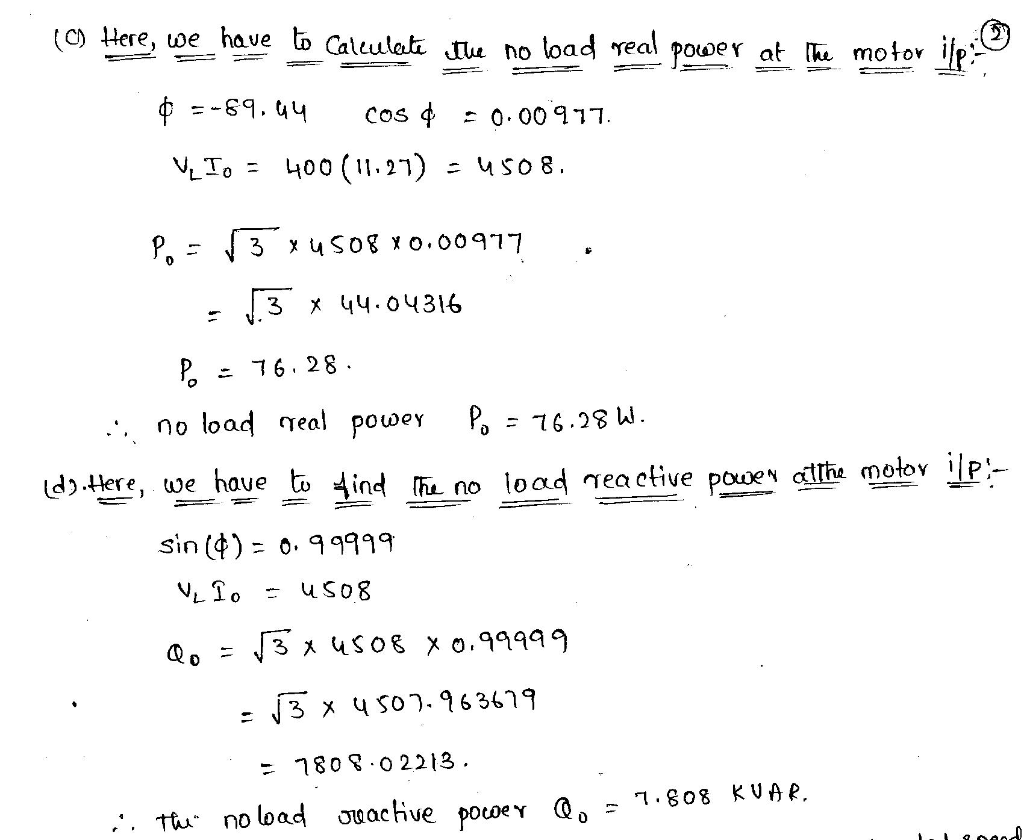NOTE:-

As per HomeworkLib rule 4 bits are enough.If you need remaining bits also please upload it as a another question.THANK YOU......

#### Earn Coins

Coins can be redeemed for fabulous gifts.

Similar Homework Help Questions
• ### Three-phase induction motor The three-phase induction motor nameplate gives the following informa...

Three-phase induction motor The three-phase induction motor nameplate gives the following information: 100 HP, 6 poles, 600 V, 60 Hz, PF =0.87. Rated motor slip s=0.0275 and rated motor current is 91.7 A. Measured at rated speed friction and ventilation losses are 1100 W. Measured between two motor terminals resistance is 0.305 Ω. f) For a load such where the motor rotates at the speed of 1180 r / min, determine shaft torque and calculate the mechanical power in HP,...

• ### A 480 V, 60 Hz, 4-pole-pair, three-phase, delta-connected induction motor has the following parameters: R1=0.42 Ω,...

A 480 V, 60 Hz, 4-pole-pair, three-phase, delta-connected induction motor has the following parameters: R1=0.42 Ω, R2=0.23 Ω, X1=0.48 Ω, X2=0.29 Ω, Xm=29.71 Ω where: R1 is the stator resistance             R2 is the rotor resistance reflected in the stator;             X1 is the stator leakage inductance;             X2 is the rotor leakage inductance reflected in the stator;             Xm is the magnetising inductance; The rotational losses are 2450 W. The motor drives a mechanical load at a speed of...

• ### A 3-phase, 208 V, 60 Hz, 30 hp, four-pole induction motor

A 3-phase, 208 V, 60 Hz, 30 hp, four-pole induction motor has the following equivalent circuit parameters. R1 = 0.2 Ω, , , Xm = 12Ω.The rotational loss is 500 W. For a slip of 5%, calculate (a) The motor speed in rpm and radians per sec (b) The current drawn by the motor from the power supply (c) The stator cu-loss (d) The air gap power (e) The rotor cu-loss (f) The shaft power (g)The developed torque and the shaft torque (h) The efficiency of the motor Use...

• ### A three-phase, six-pole 440 V, 60 Hz induction motor has the following per-phase equivalent circuit parameters...

A three-phase, six-pole 440 V, 60 Hz induction motor has the following per-phase equivalent circuit parameters referred to the stator: R1 = negligible, R’2 =0.3 Ω, Rφ = infinite, X1 = 1.131 Ω, X’2 = 1.131 Ω and Xφ = 37.7 Ω. Rotational losses are negligible. Assume the stator is connected to a 440 V, 60 Hz source and use the 6-parameters IEEE model. a) Determine the motor starting torque. (5 points) b) Calculate the motor breakdown torque. (5 points)...

• ### You want to buy three-phase asynchronous motor of 60 Hz and 480 V. You find the motor equivalent ...

You want to buy three-phase asynchronous motor of 60 Hz and 480 V. You find the motor equivalent circuit parameters (IEEE model, 6 parameters) on the manufactur-er's website: 4 poles, R1 = 0.119 Ω, R’2 =0.123 Ω, Rfe = 263 Ω, X1 = 0.683 Ω, X’2 = 0.911 Ω and Xφ = 22.8 Ω. For operation with a slip s = 1.9%, friction and ventilation losses reach 495 W. Con-sider a positive sequence balanced supply at rated voltage. Using the...

• ### The equivalent circuit of a 440 V, 3-phase, 8 pole, and 50 Hz star-connected induction motor (HELP)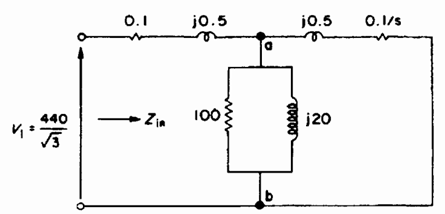Question:  The equivalent circuit of a 440 V, 3-phase, 8 pole, and 50 Hz star-connected induction motor is shown in figure 1. The short-circuit test is conducted with a locked rotor and a line current of 80 A. The open-circuit test is conducted by supplying the stator winding at rated voltage and at the rotor is rotating freely without any mechanical load.           Fig. 1 The per-phase equivalent circuit of a 3-phase induction motora)  Determine the line voltage and power factor on the...

• ### Equivalent circuit of 440V, 3-phase, 8 pole, 50 Hz star-connected induction motorThe equivalent circuit of a 440 V, 3-phase, 8 pole, and 50 Hz star-connected induction motor is shown in figure 1. The short-circuit test is conducted with a locked rotor and a line current of 80 A. The open-circuit test is conducted by supplying the stator winding at rated voltage and at the rotor is rotating freely without any mechanical load.a)     Determine the line voltage and power factor on the short circuit test;b)   We need to apply a low...

• ### 4. A certain four-pole 240-V-rms 50-Hz delta-connected three-phase induction motor operates at slip 5% at full...

4. A certain four-pole 240-V-rms 50-Hz delta-connected three-phase induction motor operates at slip 5% at full load and has rotational losses (windage + friction) of 100 W. The stator resistance per phase is 0.2 Ohm. The results of no-load and locked-rotor tests on this motor are as follows: No-load test Locked-rotor test Line-to-line input voltage: 240 V 45 V Input active power: 1100 W 1300 W Input line current: 10 A 30 A Using the tests data, determine parameters of...

• ### A two-pole, 75kW, 440 V, 50 Hz, Y-connected, three-phase squirrel cage induction motor has the fo...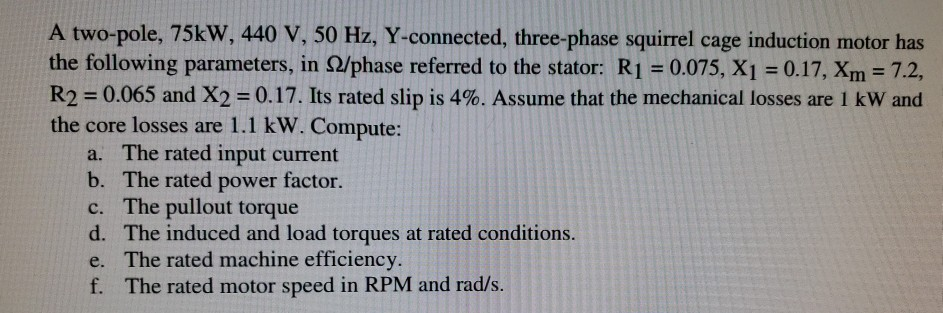Please solve this question. Thank you. A two-pole, 75kW, 440 V, 50 Hz, Y-connected, three-phase squirrel cage induction motor has the following parameters, in Ω/phase referred to the stator: R1-0.075, XI 0.17, xm-72, R2-0.065 and X-0.17. Its rated slip is 4%. Assume that the mechanical losses are 1 kW and the core losses are 1.1 kW. Compute: a. The rated input current b. The rated power factor. c. The pullout torque d. The induced and load torques at rated conditions....

• ### A three phase, 60 Hz induction motor rated at 440 V and 40 hp drives a...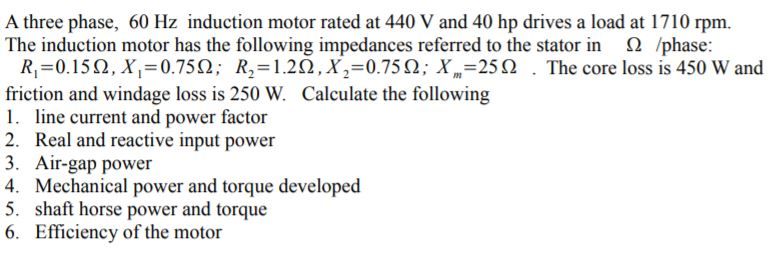A three phase, 60 Hz induction motor rated at 440 V and 40 hp drives a load at 1710 rpm. The induction motor has the following impedances referred to the stator in /phase: R,=0.1512, X,=0.7512; R2=1.222,X=0.7522; X=25 12 . The core loss is 450 W and friction and windage loss is 250 W. Calculate the following 1. line current and power factor 2. Real and reactive input power 3. Air-gap power 4. Mechanical power and torque developed 5. shaft horse...